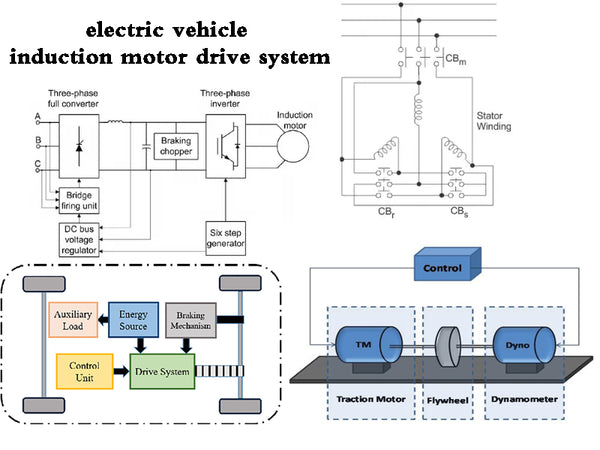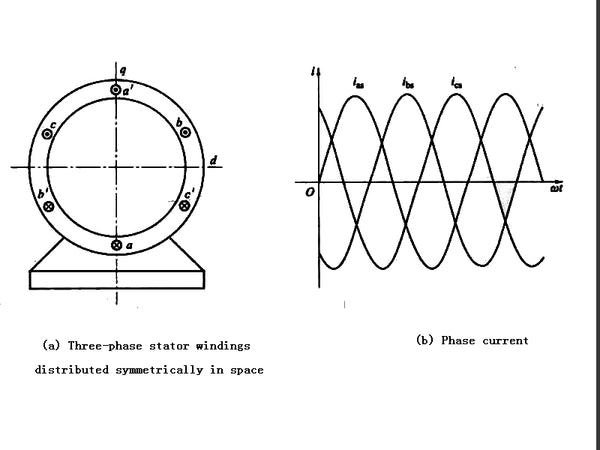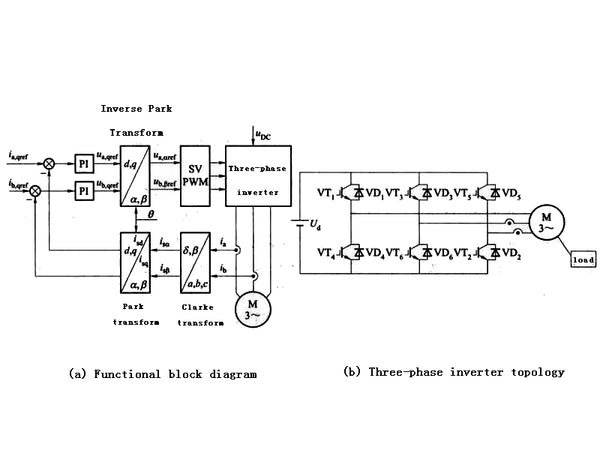Induction motor drive technology is the most mature of all brushless motor drive technologies. The rotor of the induction motor can adopt the structure with windings or the cage structure. Compared with the rotor with the cage structure, the rotor with the winding is expensive, needs maintenance and is not strong enough. Therefore, the electric drive system of the electric vehicle mostly adopts the rotor with the cage structure. The induction motor (when not strictly distinguished, the induction motor refers to the induction motor of the squirrel rotor structure). The cage rotor is composed of cast aluminum strips in the outer groove of the rotor. These cast aluminum strips are short-circuited by the cast aluminum end rings at both ends of the rotor, and the end rings can also be made into the shape of a fan.

Figure 1 shows a schematic diagram of the stator and stator winding currents of a three-phase, two-pole induction motor. The stator magnetomotive force composite vector rotates at an angular velocity ω. The interaction between the rotating stator magnetomotive force and the rotor conductors will induce a voltage, and thus a current, in the rotor. The rotating magnetomotive force then produces torque on the rotor carrying the induced current. Obviously, the induced current in the rotor is necessary to generate torque, and likewise, the induced current also depends on the relative motion between the stator magnetomotive force and the rotor. This is also why there must be a difference between the angular velocity of the rotating stator magnetomotive force and the angular velocity of the rotor. The slip s is defined as
s=(ωmsm)/ωms

When s>0, the motor works in motor mode and generates stable torque; when s=0, no torque is generated; when s<0, the motor runs in a power-generating state, generating negative torque.Figure 1 Schematic diagram of the stator and stator winding currents of a three-phase, two-pole induction motor

Due to the coupling of AC and DC in the induction motor model, its dynamic model is a multi-variable, strong coupling, nonlinear model, and the speed control of induction motor is much more complicated than that of DC motor. Common control strategies are variable-voltage variable-frequency (VVVF) control, field-oriented control (FOC), also called vector control or decoupling control, direct-torque control (direct-torque control, DTC). These control strategies also have some complex control algorithms, such as adaptive control, variable structure control, optimization control, etc., to obtain faster response speed, higher efficiency and wider working range.

The VVVF control strategy adopts constant voltage/chin ratio control when the frequency is lower than the rated operating frequency of the motor, and adopts constant voltage variable frequency control when the frequency is higher than the rated frequency. VVVF control is rarely used in high-performance induction motor drive systems due to fluctuating air-gap flux and slow response, and low power factor resulting in low operating efficiency.

At present, the control strategies used in high-performance induction motor systems mainly include vector control and direct torque control. Their theoretical basis is derived from the multivariate mathematical model of the motor. Vector control technology was proposed by Blaschke et al. in Germany in 1971. Its basic idea is derived from the strict simulation of DC motors. The stator current vector is divided into excitation component and torque component through the orientation of the motor magnetic field, and they are controlled separately to obtain good performance. decoupling properties. Direct torque control was proposed by Takahashi in Japan and M. Depenbrock in Germany in the 1980s respectively in the induction motor theory. Direct control of the flux linkage. Direct torque control has the advantages of simple structure, small dependence on parameters, and fast dynamic response. Table 1 shows the system performance comparison of these two control methods.

 Compare items Vector control Direct torque control Inverter switching frequency High Low Torque ripple Small Big Speed range Wide range Limited range Low speed performance Good Serious torque ripple Current Distortion and Harmonics Generally Big the complexity of the system Generally Simple Sensitivity of parameters Generally Small Startup performance Good, soft start Poor, big start shock

Table 1 Comparison of system performance of two control methods

By comparing the system performance of the two control methods in Table 1, it can be concluded that vector control is a more mature control technology than direct torque control, and has been more widely used in AC induction motor systems. The theory of direct torque control still needs to be further perfected, and its problems in low-speed performance, torque ripple, motor starting, etc. need to be solved. Therefore, the control scheme of the induction motor system generally chooses the vector control scheme.

Coordinate transformation is essential for vector control systems. The Clarke transformation is used to realize the conversion of the system from the natural axis system (abc axis system) to the two-phase stationary axis system (α, β axis system). The coordinate system, stator current vector and its components are shown in Figure 2(a). Park transformation realizes the transformation between the two-phase stationary shaft system (α, β shaft system) to the two-phase rotating shaft system (d, q axis system). Its coordinate system, stator current vector and its components are shown in Figure 2(b). As shown, the transformation from the d and q axes to the α and β axes is the inverse Park transformation.Figure 2 Stator current space vector and its components

The direction of the rotor flux linkage vector is selected as the coordinate horizontal axis d, and the dq reference coordinate system rotates synchronously with the rotor magnetic flux, and the electromagnetic torque of the motor can be expressed as
Te=3/2·p·(Lm/Lr)·iqs·λdr
In the formula: p is the number of pole pairs;
Lm—armature inductance of each phase;
Lr——rotor inductance of each phase;
iqs - the q-axis component of the stator current;
λdr—d-axis component of rotor flux.

This is similar to the torque formula of the separately excited DC motor, which can keep the magnetic field component λdr unchanged and control the torque component iqs to adjust the motor torque output, so that the induction motor can obtain the required fast dynamic response. The coordinate transformation makes the induction motor obtain a control method similar to that of the DC motor, which is also the basis of the unified theory of the motor.

The structure of the induction motor vector control system is shown in Figure 3. The two phases of the three-phase stator current are measured and sent to the Clarke module. The outputs i and i are used as the input of the Park transformation module to obtain the excitation component isd and torque component isq in the dq rotating coordinate system, which are respectively related to the stator current reference components isdref and isqref Compared. Since the rotor magnetic flux in the PMSM of the permanent magnet synchronous motor is generated by the permanent magnet, it is a fixed value, so the rotor excitation component is not required, that is, isdref=0. The system structure shown in Figure 3 can be used for PMSM drive system control. If speed space vector control is used, isqref can also be the speed regulator output.Figure 3 Structure of induction motor vector control system

In a synchronous motor, the rotor speed is equal to the rotor flux speed, and the rotor position angle can be directly measured by the position sensor or obtained by integrating the rotor speed. In an induction motor, the rotor speed is not equal to the rotor flux speed (with slip), and a special method is needed to calculate the rotor position angle. The basic method is to use the current model to establish two equations of the motor. SVPWM produces the smallest current harmonic distortion in the three-phase AC motor windings, and the space voltage vector modulation technique is more efficient in utilizing the supply voltage than the sinusoidal modulation method.